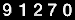⇐     slow      fast     ⇒

Your browser does not support the HTML5 canvas tag.

Click the upper left corner to choose a state
The animation shows the quantum noise of the electric field of different freely propagating light waves (left) and the corresponding moving wave packet in the harmonic potential (right).
The unit of the quantum noise amplitude is the same as the one of the wave packets x-axis: 1 unit corresponds to the quantum noise of the vacuum state.
The first thirteen states were measured at the University of Konstanz. The following one, the star state, was a computer simulation performed by Timo Felbinger in Konstanz. The measurement time of the quantum noise depended on the (experimentally adjustable) phase change of the detection system. It corresponds to the time development of the light wave. For the first ten states a typical measurement time of one data set was 200 ms. To be able to distinguish single points of the noise data only 10.000 that is 1/50 of the original 512k data points are plotted. For the experimental photon statistics, bars represent the theoretical expectation, black dots the experimental values.

The experimental data are followed by online calculations, depending on the parameter input you choose. Altogether you can generate so far:
• Coherent States
• Squeezed States
• Thermal States and Squeezed Thermal States
• Coherent Superposition of two Coherent States (Schrödinger Cat State)
• Number States
• Coherent Superposition of a Number State with a Coherent State (Schrödinger Cat State by quantum optical catalysis)
• Coherent Superposition of two Number States
• Synthesis of a Coherent State by stepwise Addition of Number States
• Synthesis of a Fock State (Number State) by stepwise Addition of Coherent States
• Coherent Displacement of a Fock State
to be continued...

START - starts the animation
WIGNER - shows the corresponding phase space distribution function
PHOTON - shows the corresponding photon statistics

The Wignerfunction ist calculated via Invers Radon Transform using as a filter function a quadratic regularization.
The Photon Number Distribution ist calculated by the Leonhardt-d'Ariano-Algorithm, the input "Max Photons" gives the limit up to which n the density matrix in the number state representation is internally calculated. In principle the program provides the whole density matrix, but so far the graphical output has still some limitations.

The second part of the choices provides facilities to generate all kinds of simulated states of the light field.
The "Coherent Superpositions of two Coherent States" demonstrates strikingly the difference between classical and quantum mechanical superposition / interference of waves.
The "Coherent Superpositions of a Number State with a Coherent State" elucidates some background of the Lvovsky experiments.
The "Coherent Superpositions of two Number States" and "Synthesis of Coherent States" follows the path of Schrödingers original thinking, how it is possible to form wave functions that change in time out of stationary states. The synthesis of coherent states corresponds to plain Fourier Analysis.
The "Synthesis of Fock States (Number States) by stepwise Addition of Coherent States" is kind of the Inverse transform of the "Synthesis of Coherent States". The challenging point though is that the coherent states form an overcomplete set as a basis of the underlying Hilbert Space. Therefore there are several ways to build Number States out of Coherent States. In the Animation above you have to reduce the Amplitude more and more to approach the Number States. Two interfering "almost vacua" generate a One-Photon-State! Three interfering "almost vacua" generate a Two-Photon-State and so on. If the amplitudes of the interfering states are equal or assume the limit 0 then nothing remains! So the limit of the state is not reached by plugging in the limit of the parameter!
If and independently, then
+    and
+ +